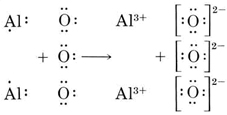6.09: The Octet Rule

Because binary ionic compounds are confined mainly to group 1 and group 2 elements on the one hand and group VI and VII elements on the other, we find that they consist mainly of ions having an electronic structure which is the same as that of a noble gas. In calcium fluoride, for example, the calcium atom has lost two electrons in order to achieve the electronic structure of argon, and thus has a charge of +2:1s22s22p63s23p64s2 → 1s22s22p63s23p6 + 2e

By contrast, a fluorine atom needs to acquire but one electron in order to achieve a neon structure. The resulting fluoride ion has a charge of –1:

The outermost shell of each of these ions has the electron configuration ns2np6, where n is 3 for Ca2+ and 2 for F. Such an ns2np6 noble-gas electron configuration is encountered quite often. It is called an octet because it contains eight electrons. In a crystal of calcium fluoride, the Ca2+ and F ions are packed together in the lattice shown below. Careful study of the diagram shows that each F ion is surrounded by four Ca2+ ions, while each Ca2+ ion has eight F ions as nearest neighbors.

Thus there must be twice as many F ions as Ca2+ ions in the entire crystal lattice. Only a small portion of the lattice is shown, but if it were extended indefinitely in all directions, you could verify the ratio of two F for every Ca2+. This ratio makes sense if you consider that two F ions (each with a –1 charge) are needed to balance the +2 charge of each Ca2+ ion, making the net charge on the crystal zero. The formula for calcium fluoride is thus CaF2.Figure $$\PageIndex{1}$$ A portion of the ionic crystal lattice of fluorite, calcium fluoride. (a) Ca2+ ions (color) and F– ions (gray) are shown full size. “Exploded” view shows that each F– surrounded by four Ca2+ ions, while each Ca2+ ion is surrounded by eight F– ions. The ratio of Ca2+ ions to F– ions is thus 4:8 or 1:2, and the formula is CaF2. (Computer-generated). (Copyright © 1976 by W. G. Davies and J. W. Moore.)

Newcomers to chemistry often have difficulty in deciding what the formula of an ionic compound will be. A convenient method for doing this is to regard the compound as being formed from its atoms and to use Lewis diagrams. The octet rule can then be applied. Each atom must lose or gain electrons in order to achieve an octet. Furthermore, all electrons lost by one kind of atom must be gained by the other.

An exception to the octet rule occurs in the case of the three ions having the He 1s2 structure, that is, H, Li+ and Be2+. In these cases two rather than eight electrons are needed in the outermost shell to comply with the rule.

Example $$\PageIndex{1}$$: Ionic Formula

Find the formula of the ionic compound formed from O and Al.

Solution: We first write down Lewis diagrams for each atom involved:We now see that each O atom needs 2 electrons to make up an octet, while each Al atom has 3 electrons to donate. In order that the same number of electrons would be donated as accepted, we need 2 Al atoms (2 × 3e donated) and 3 O atoms (3 × 2e accepted). The whole process is thenThe resultant oxide consists of aluminum ions, Al3+, and oxide ions, O2–, in the ratio of 2:3. The formula is Al2O3.